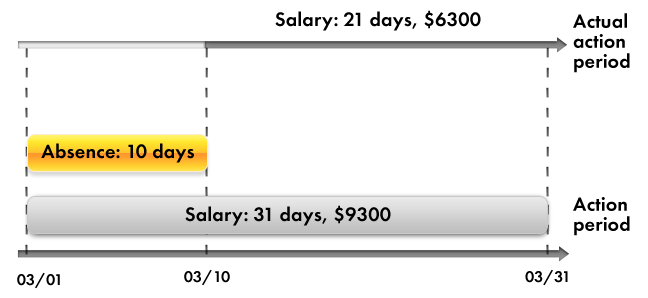# Displacement by action period

A calculation type can influence the calculation period of another calculation type instead of its source data.

As an example, consider salary calculation with absences taken into account. Suppose that you calculate an employee's salary for March. In this case the action period for the calculation is from 3/1/2014 to 3/31/2014.

Then a manager informs you that the employee was absent from the 1st to the 10th of March for some unknown reason. So you need to perform the Absence calculation, which might introduce some deductions from the payment.

But you also need to recalculate the employee's salary based on the fact that the actual Salary calculation period is now from 3/11/2014 to 3/31/2014.

We will refer to this influence as the displacement by action period.

As a result, if the employee would have received \$9,300 for a full month work, the accrual for the actual period comes to \$6,300 (fig. 17.2).Fig. 17.2. A record of the Absence calculation displaces a record of the Salary calculation by action period

Thus, based on the two types of mutual influence of calculations, one could come to a conclusion that in general there are three periods associated with each calculation type: action period, actual period, and base period.

• Action period is a requested period. This means that when you specify an action period, your request is "I would like the result to be valid for this period."
• Actual period is what remains after analyzing all the calculation action periods that displace your calculation by action period.
• Base period is the period within which you analyze the results of other calculations that influence your calculation by that period.

As you see, the mutual influence of calculation types can vary greatly and can be multilayered, which complicates everything the most. In other words, one calculation type might influence another, which in turn affects a third one, and so on.

Obviously, this situation calls for some kind of universal mechanism that would allow you to define each of these calculation types (its algorithm, influences on other calculation types, and dependencies), to store data obtained as a result of these calculations, and to monitor the need to recalculate the results of dependent calculations upon changes in the results of "primary" calculations.

Within 1C:Enterprise this universal mechanism is implemented using charts of calculation types and calculation registers.

The first configuration object we will discuss during this lesson is the Chart of calculation types.

Be the first to know tips & tricks on business application development!